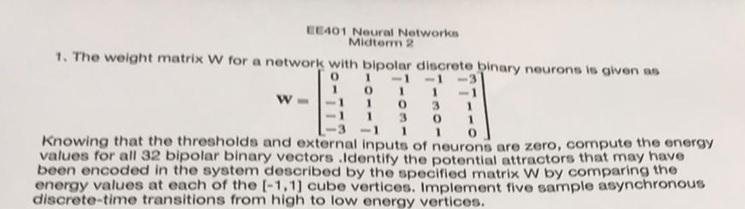# (Solved): 1. The weight matrix $$W$$ for a network with bipolar discrete binary neuro ...???????

1. The weight matrix $$W$$ for a network with bipolar discrete binary neurons is given as $\mathbf{W}=\left[\begin{array}{ccccc} 0 & 1 & -1 & -1 & -3 \\ 1 & 0 & 1 & 1 & -1 \\ -1 & 1 & 0 & 3 & 1 \\ -1 & 1 & 3 & 0 & 1 \\ -3 & -1 & 1 & 1 & 0 \end{array}\right]$ Knowing that the thresholds and external inputs of neurons are zero, compute the energy values for all 32 bipolar binary vectors. Identify the potential attractors that may have boon oncoded in the system describod by the specified matrix $$\mathrm{W}$$ by comparing the onergy values at each of the $$[-1,1]$$ cube vertices. Implement five sample asynchronous discrete-time transitions from high to low energy vertices.

We have an Answer from Expert